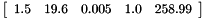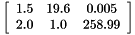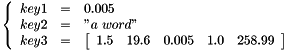String representation of values

OpenFLUID values can be converted into strings, using the following representations

# Simple values

Representation of simple values is trivial. In OpenFLUID, it is based on classical string representations.

## BooleanValue

Values of BooleanValue type are converted into the true or false string.

## IntegerValue

Values of IntegerValue type are converted into their textual representation. As an example, the value 192 will be converted to the 192 string.

## DoubleValue

Values of DoubleValue type are converted into their textual representation. As an example, the value 17.37 will be converted to the 17.37 string.

## StringValue

Since values of StringValue type are natively stored as string, they are not converted and represented as they are.

# Compound values

Representation of compound values requires a more complex representation schema. In OpenFLUID, it is based on the JSON data format without any space or newline.

## VectorValue

Values of VectorValue type are converted using the JSON notation for vectors which is a comma separated list of values enclosed by an opening square bracket and a closing square bracket.

As an example, the following vectorwill be converted into the [1.5,19.6,0.005,1.0,258.99] string.

## MatrixValue

Values of MatrixValue type are converted using the JSON notation for matrix which are considered as a vector of vector(s).

As an example, the following matrixwill be converted into the [[1.5,19.6,0.005],[2.0,1.0,258.99]] string.

## MapValue

Values of MapValue type are converted using the JSON notation for objects which is a comma separated key-value list enclosed by an opening curly bracket and a closing curly bracket.

As an example, the following mapwill be converted into the {"key1":0.005,"key2":"a word","key3":[1.5,19.6,0.005,1.0,258.99]} string.

## TreeValue

The string format for TreeValue is not stable and will be updated in further versions to match the philosophy of string formats for other compound OpenFLUID values.